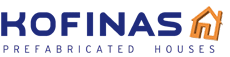# Complex of two D-115, total surface of 2 x 115,20 = 230,40 m²

Complex of two 2-story houses D-115 with total surface of 2 x 115,20 = 230,40 m²

# Complex of 1-story houses E-25b, total surface of 4 x 25,85 = 103,40 m²

House complex of total surface of 4 x 25,85 = 103,40 m²

# Complex of 1-story houses E-62b, total surface of 3 x 62,63 = 187,89 m²

House complex of total surface of 3 x 62,63 = 187,89 m²

# Complex of 1-story houses with attics E-63, total surface of 3 x 63,35 = 190,05 m²

House complex of total surface of 3 x 63,35 = 190,05 m²

# Complex of 1-story houses E-40a, total surface of 4 x 40,10 = 160,40 m²

House complex of total surface of 4 x 40,10 = 160,40 m²

# Complex of 2-story houses E-62, total surface of 4 x 62,60 = 250,40 m²

House complex of total surface of 4 x 62,60 = 250,40 m²

# Complex of 1-story houses E-25a, total surface of 4 x 25,85 = 103,40 m²

House complex of total surface of 4 x 25,85 = 103,40 m²

# Complex 3-story houses E-84, total surface of 4 x 84,17 = 336,68 m²

Complex of four 3-story houses T-83 with total surface of 4 x 84,17 = 336,68 m².

# Complex of houses E-136, total surface of 3 x 136,39 = 409,17 m²

Complex of three 2-story houses D-136 with total surface of 3 x 136,39 = 409,17 m².

# Complex of 1-story houses E-40b, total surface of houses 8×40,04 & staircases 4×7,70 = 351,12 m²

House complex of total surface of houses 8×40,04 & staircases 4×7,70 = 351,12 m²519 Mesogeion Avenue, Aghia Paraskevi, Attika
T: +30 210 6081307
F: +30 210 6016177
[email protected]